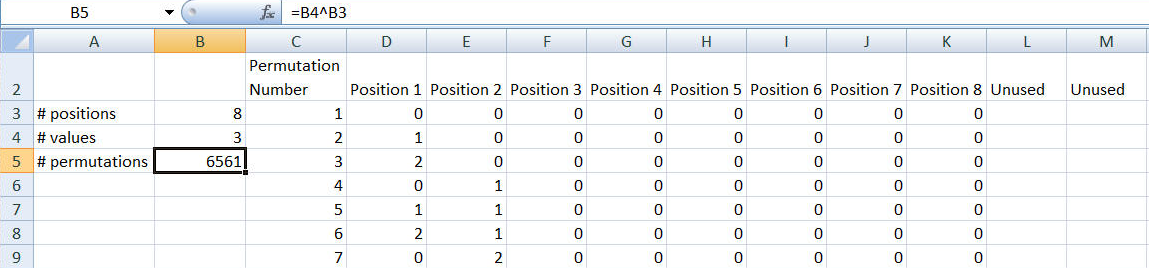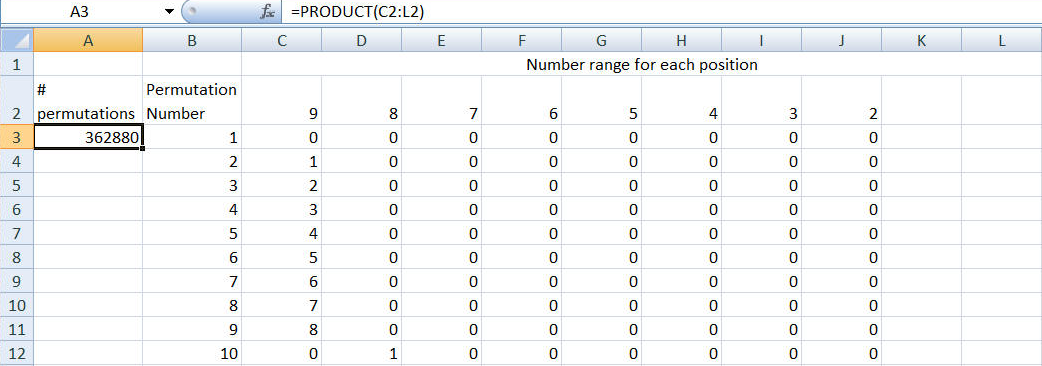You are on the Home/Publications & Training/Case Studies/Generate All Permutations pageShare Your

# Generate All Permutations

This tip documents how to generate all the permutations under two different scenarios.

In the first scenario, there are N identical dice, each with M sides, with each side uniquely labeled from 0 to M-1. So, a 6 sided dice would have the numbers 0 to 5 and a tetrahedral (four sided) die would have the numbers 0 to 3.

In the second scenario, each of the N die has a different number of sides. This scenario might apply to, say, all the possible permutations that one can get from a car model with M trims, N tire choices, P interiors, and Q colors.

## All Permutations from Identical Choices

The idea here is to have one die correspond to one column. Each row will have one possible permutation, starting with zero. The formula in this column will compute one number from the previous one with the formula =MOD({previous number} +1, {number of choices}). So, for a six-sided dice, we will get 0, 1, 2, 3, 4, 5, 0. The next column over will use a slightly modified formula. It will retain the current value until the previous column rolls over to zero. When that happens, the column will use the same MOD() formula to calculate its next number. So, it will go 0, 0, 0, 0, 0, 0, 1. Each of the however many columns we add will use the same approach, making this solution easily scalable. Since each row holds one permutation, the total number of rows required will be MN. With this background, we can implement the solution in Excel.The number of dice (positions) is in B3, the number of sides on each die (the range of values for each position) is in B4. B5 gives the total number of permutations as =B4^B3.

The column headings (row 2 starting with D2) are created with the formula =IF(COLUMN()-COLUMN(\$C\$2)<=\$B\$3,"Position "&(COLUMN()-COLUMN(\$C\$2)),"Unused")

Column C sequentially numbers each permutation. C3 contains the formula =IF(ROW()-ROW(\$D\$3)+1<=\$B\$5,1,"") and C4 contains =IF(ROW()-ROW(\$D\$3)+1<=\$B\$5,C3+1,"").

D3 contains the formula =IF(C3<>"",IF(COLUMN()-COLUMN(\$D\$3)+1<=\$B\$3,0,""),"") and E3=IF(D3="","",IF(COLUMN()-COLUMN(\$D\$3)+1<=\$B\$3,0,"")). E3 is copied over to F3:M3.

D4 contains =IF(C4<>"",MOD(D3+1,\$B\$4),"") and E4 contains =IF(D4="","",IF(AND(D3<>D4,D4=0),MOD(E3+1,\$B\$4),E3)).  E4 is copied over to F4:M4.

C4:M4 are copied down until column C lists the final permutation (i.e., the one with the same number as in B5).  One can also make sure that at least one row contains all blanks.

## All Permutations from Non-identical Choices

With the above solution, it is relatively easy to figure out how to get all the permutations for the scenario where each die has a possibly different number of sides.  Instead of the divisor of the MOD operation being the same number (B4 in the above example), we use a different divisor for each die.  The rest of the solution remains the same!Row 2 lists the number of choices possible for each column.  In the above example, they range from 9 in position 1 (column C) to 2 in position 8 (column J). The total number of permutations is given by the product of all the individual ranges. A3 contains the formula =PRODUCT(C2:L2).

The sequential numbering of the permutations is in column B.  B3 contains the formula =IF(ROW()-ROW(\$B\$3)+1<=\$A\$3,1,"") and B4 contains =IF(ROW()-ROW(\$B\$3)+1<=\$A\$3,B3+1,"").

For the first permutation (all zeros), in C3 enter the formula =IF(C2<>"",0,"") and copy C2 to D2:L2.

The subsequent permutations are calculated as follows: In C4 enter the formula =IF(B4<>"",MOD(C3+1,C\$2),""). In D4 enter =IF(C4="","",IF(AND(C3<>C4,C4=0),MOD(D3+1,D\$2),D3)).  Copy D4 to E4:L4. Finally, copy B4:L4 as far down as necessary to get the permutation numbered the same as the value in A3. Alternatively, ensure that at least one row contains all blanks.